# Team:XMU-China/modeling

XMU-CSS

XMU

modelingindex

Contents[hide][show]
• Introduction
• Modeling
• Result
• Reference
• Model

Modeling

1. Introduction
Ordinary differential equation(s) (ODE) is one of the most popular methods in modeling. Frank R. Giordano and other scientists have introduced it exhaustively.  In many computational biological researching, researchers often use it to simulate the dynamics part of biological process. The concentrations of RNA, proteins, and other molecules are represented by time-dependent variables.  We used the same method to construct our model.

2. Modeling
First of all, there are 4 variables and 4 parameters in this experience. Their names and meanings are listed below.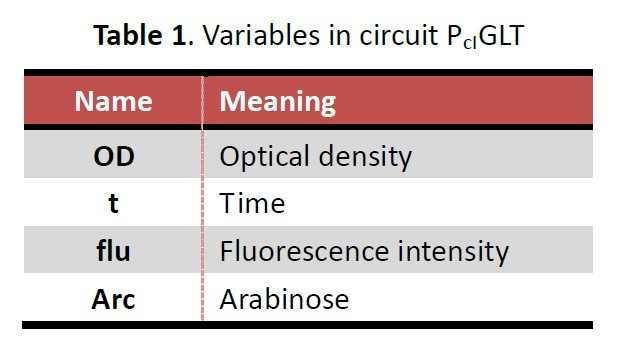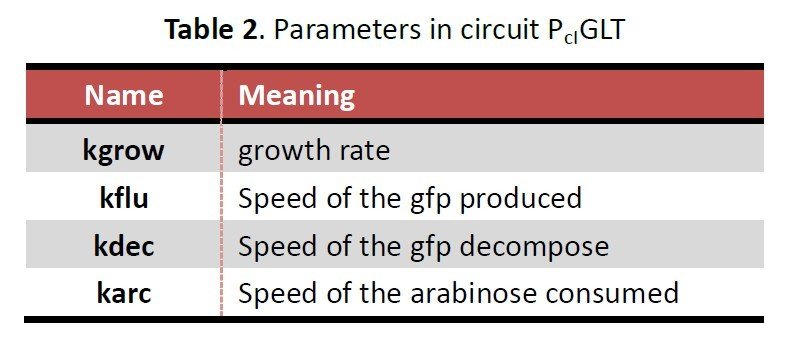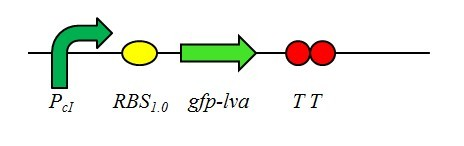Those functions about describing the rate equations of biochemical reactions in the circuit PcIGLT are: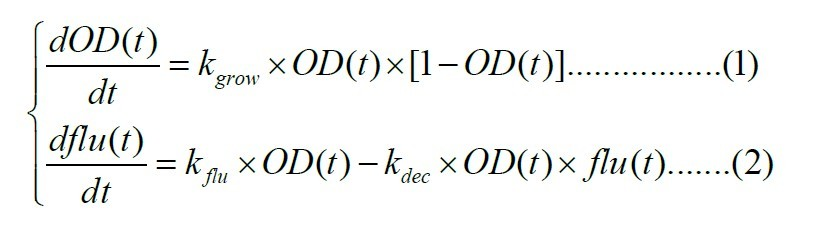The initial condition is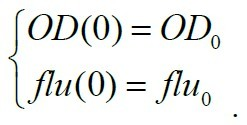Equation (1) represents the course of growth of E.coli. Equation (2) represents the course of producing and decomposing GFP. OD0 and flu0 is value of OD and flu when t=0. Then, we changed the parameters and figured out the best value for fitting the data of fluorescence intensity.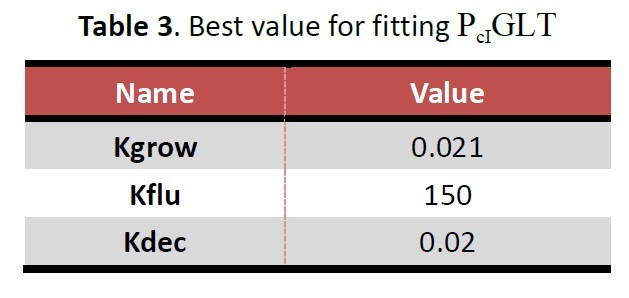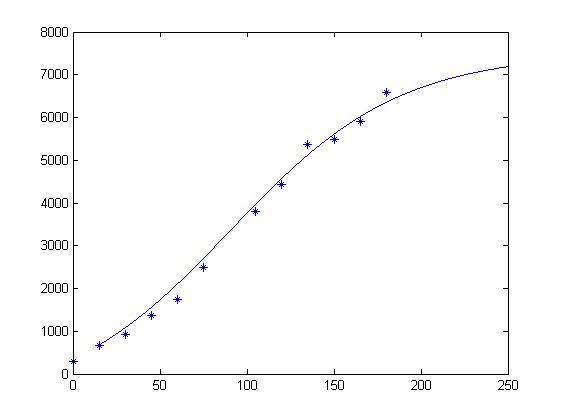Figure 1: Fitting line and data of fluorescence intensity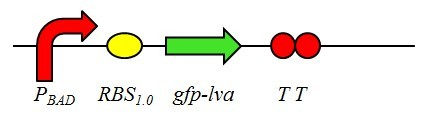Those functions about describing the rate equations of biochemical reactions in the circuit PcIGLT are: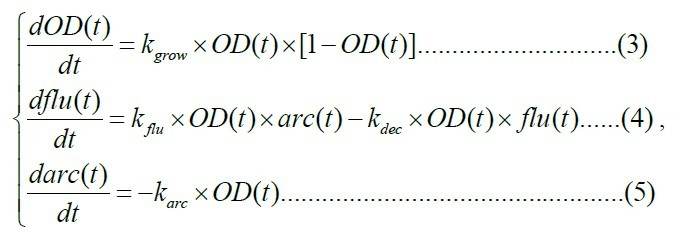The initial condition is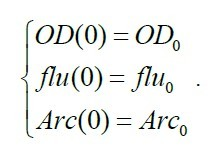Equation (3) represents the course of growth of E.coli.. Equation (4) represents the course of producing and decomposing GFP. Equation (5) represents the arabinose utilized for inducing. OD0, flu0 and Arc0 is value of OD, flu and Arc when t=0. After that, we changed the parameters and then found out the value fitting the data of fluorescence intensity most.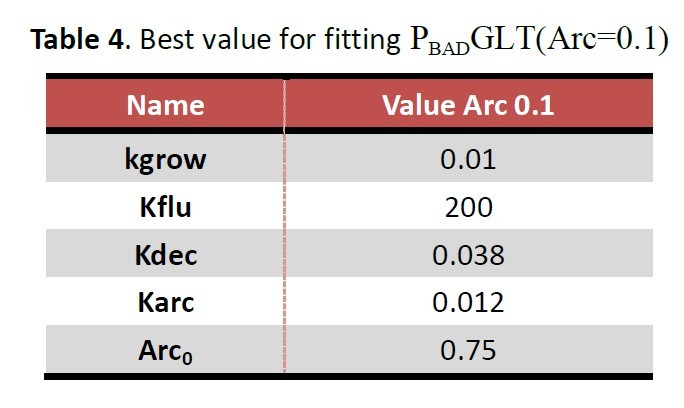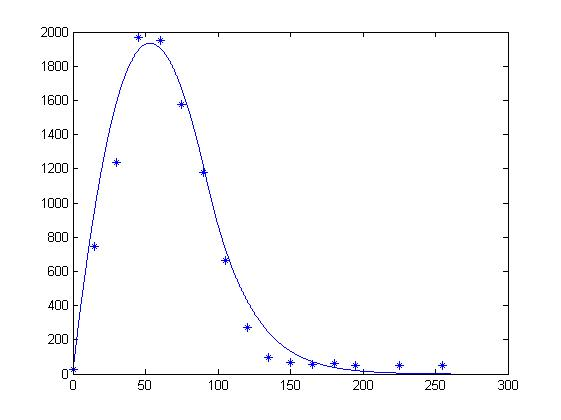Figure 2: Fitting line and data of fluorescence intensity (Value Arc=0.1).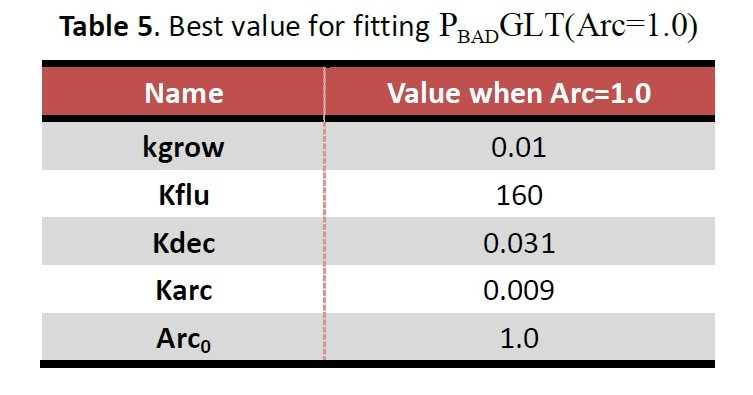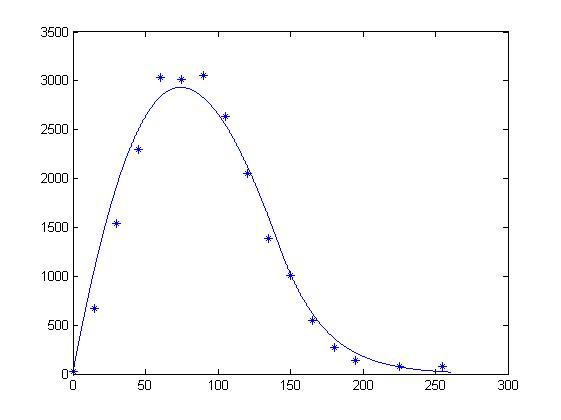Figure 3: Fitting line and data of fluorescence intensity (Value Arc=0.1).

3. Result
According to Figure (1-3), we can draw a conclusion that the model we constructed can simulate the process of dynamic change in fluorescence intensity. We can figure out how those parameters work in this model if we have more experience data.

4. Reference